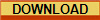Publication History: This article is based on Chapter 10 of "The Log Analysis Handbook" by E. R. Crain, P.Eng., published by Pennwell Books 1986  Updated 2004. This webpage version is the copyrighted intellectual property of the author. Do not copy or distribute in any form without explicit permission.PERMEABILITY from Dumanoir and Coates
The original model is somewhat complicated and not very accurate in water bearing zones due to a number of assumptions that need to be made. The simplofied model is popular because it treats the porosity in the same way as the dual-water saturation model. Both total and effective porosity are used, so there is an implicit shale correction to the permeability calculation.

First find the resistivity log reading in the irreducible water zone:
1: IF Sw > 0.70
2: THEN SWir = KBUCKL / PHIe
3: AND RESir = A * RW@FT / (PHIe ^ M) / (SWir ^ N)
4: OTHERWISE RESir = RESD

Then calculate the porosity exponent (LPERM)
5: LPERM = (3.75 - PHIe + ((log (RW@FT / RESir) + 2.2) ^ 2) / 2) ^ 0.5

And finally:
6: PERMd = LPERM * ( 0.077 + 1.55 * DENShy - 0.627 * DENShy ^ 2)
* (PHIe ^ (2 * LPERM)) / ((LPERM ^ 4) * (RW@FT / RESir))) ^ 2

Where:
A = tortuosity exponent (fractional)
KPERM = permeability constant (fractional)
LPERM = porosity exponent (fractional)
DENShy = hydrocarbon density (gm/cc)
M = cementation exponent (fractional)
N = saturation exponent (fractional)
PERMd = calculated permeability (millidarcies)
PHIe = effective porosity (fractional)
KBUCKL = porosity saturation product (fractional)
RESD = resistivity log reading (ohm-m)
RESir = resistivity at irreducible water saturation (ohm-m)
RW@FT = water resistivity at formation temperature (ohm-m)
Sw = water saturation (fractional)
SWir = irreducible water saturation (fractional)COMMENTS:
Reference:
1. A New Approach to Improved Log Derived Permeability,
G.R. Coates, J.L. Dumanoir, SPWLA, 1973.

This complex formula does not lend itself to graphical solution, although some charts are provided in the original technical paper. A simpler version of this algorithm can be found below.RECOMMENDED PARAMETERS:
KPERM is usually set at 90,000, but can be modified with local experience.NUMERICAL EXAMPLE
Assume data from Classic Example Sand B.
PHIe = 0.30
RW@FT = 0.02 ohm-m
A = 0.62
M = 2.15
SWir = Sw = 0.25
RESD = 20 ohm-m
N = 2.00
DENShy = 0.8 gm/cc

Dumanoir and Coates method:
KPERM = 90,000
LPERM = (3.75 - 0.30 + ((log (0.2 / 20) + 2.2) ^ 2) / 2) ^ 0.5 = 1.86
PERMd = 90,000*(0.077+1.55*0.8-0.627*0.8*0.8)*(0.3^(2*1.86)) / ((1.86^4)*(0.2/20)))^2
PERMd = 90,000 * (0.915 * 0.0Permeability From the Coates Simplified MethodPERMc - Permeability From the Coates Simplified Method
This is a simplification of an earlier method proposed by Dumanoir and Coates. It is more optimistic than other methods in low porosity.
7: PERMc = GPERM * PHIe ^ 4 * ((PHIt - PHIe * SWir) / (PHIe * SWir)) ^ 2

OR in clean zones:
8: PERMc = GPERM * PHIe ^ 4 * ((1 - SWir) / SWir) ^ 2

Where:
GPERM = permeability scale factor (fractional)
PERMc = calculated permeability (millidarcies)
PHIe = effective porosity (fractional)
PHIt = total porosity (fractional)
SWir = irreducible water saturation (fractional)COMMENTS:
This method works well in shaly sands. As the difference between PHIt and PHIe increases, PERMc decreases.RECOMMENDED PARAMETERS:
GPERM = 6500 to 10000 for oil, 650 to 1000 for gas"META/PERM"  Compare Permeability Calculated from Various MethodsPage Views ---- Since 01 Jan 2015